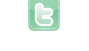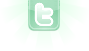## Getting random choice without repetition

I have copied the code from this Topic. I used the exact one for different mechanism. But how will I write if it the number of classes to be added were 5 instead of 3
Roshan123 wrote...
I have copied the code from this Topic. I used the exact one for different mechanism. But how will I write if it the number of classes to be added were 5 instead of 3
Basically:
```// with fields
private Object[] choices = { choiceA, choiceB, choiceC, choiceD, choiceE, ... };  // any number of choices
private int previousChoice = -1; // index for 'choices' array; '-1' = none chosen yet

// to make choice
int rand = Greenfoot.getRandomNumber(choices.length - prreviousChoice < 0 ? 0 : 1); // choosing one
if (previousChoice >= 0 && rand >= previousChoice) rand++; // avoid previous (choice num to index num)
Object obj = choicees[rand]; // new choice
previousChoice= rand; // update last choice```
danpost wrote...
Basically:
```// with fields
private Object[] choices = { choiceA, choiceB, choiceC, choiceD, choiceE, ... };  // any number of choices
private int previousChoice = -1; // index for 'choices' array; '-1' = none chosen yet

// to make choice
int rand = Greenfoot.getRandomNumber(choices.length - prreviousChoice < 0 ? 0 : 1); // choosing one
if (previousChoice >= 0 && rand >= previousChoice) rand++; // avoid previous (choice num to index num)
Object obj = choicees[rand]; // new choice
previousChoice= rand; // update last choice```
Got it!!! Thanks for explaining the code well